## Example Questions

← Previous 1

### Example Question #81 : Graphing

In the standard xy coordinate plane, what quadrant(s) could point A lie in, if its x-coordinate and y-coordinate have opposite signs?

Explanation:

We know that right of the origin represents the positive x-direction, and above the origin represents the positive y-direction.  For Quadrant I, both the x-coordinate and y-coordinate must be positive, i.e. (+,+).  For quadrant II, the x-coordinate is negative, while the y-coordinate is positive, i.e. (+, -). For Quadrant III, both coordinates must be negative, i.e. (-, -).  For Quadrant IV, the x-coordinate is positive, and the y-coordinate is negative, i.e. (+, -).

### Example Question #2 : How To Graph An Ordered Pair

Which pair of coordinates lies within quadrant II?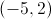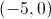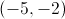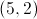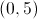Explanation:

By definition, quadrant I has positive coordinates, quadrant II has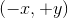coordinates, quadrant III has negative coordinates, and quadrant IV has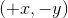coordinates, as shown by the diagram below. Only one answer choice falls within quadrant II. All others lie in other coordinates or one of the axes.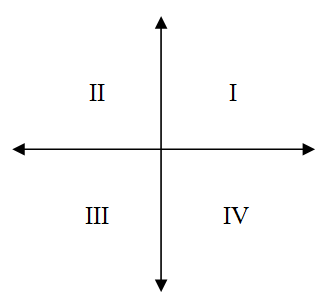- Quadrant II- Quadrant I- x-axis- y-axis- Quadrant III

### Example Question #231 : Coordinate Plane

In which Quadrant is the point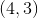located?

Explanation:

To find out which quadrant a point lives in, we need to remember the qualities of each quadrant. By qualities, I mean the signs associated with the x and y values which are dependent on the quadrant the point resides in.

All coordinate pairs that are in Quadrant I will have a positive x value and a positive y value.

All coordinate pairs that are in Quadrant II will have a negative x value and a positive y value.

All coordinate pairs that are in Quadrant III will have a negative x value and a negative y value.

All coordinate pairs that are in Quadrant IV will have a positive x value and a negative y value.

For the point,  we see that both the x and y coordinates are positive therefore, the point resides in Quadrant I. Another way to check, we can start from the origin and going right 4 units and up 3 units we will be in the upper-right section of the coordinate-plane, which is also known as Quadrant I.

### Example Question #1 : How To Graph An Ordered Pair

Which of the following coordinate pairs is farthest from the origin?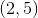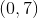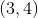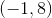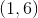Explanation:

Using the distance formula, calculate the distance from each of these points to the origin, (0, 0). While each answer choice has coordinates that add up to seven, (-1, 8) is the coordinate pair that produces the largest distance, namely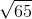, or approximately 8.06.

### Example Question #1 : How To Graph An Ordered Pair

Which point best represents the coordinate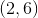?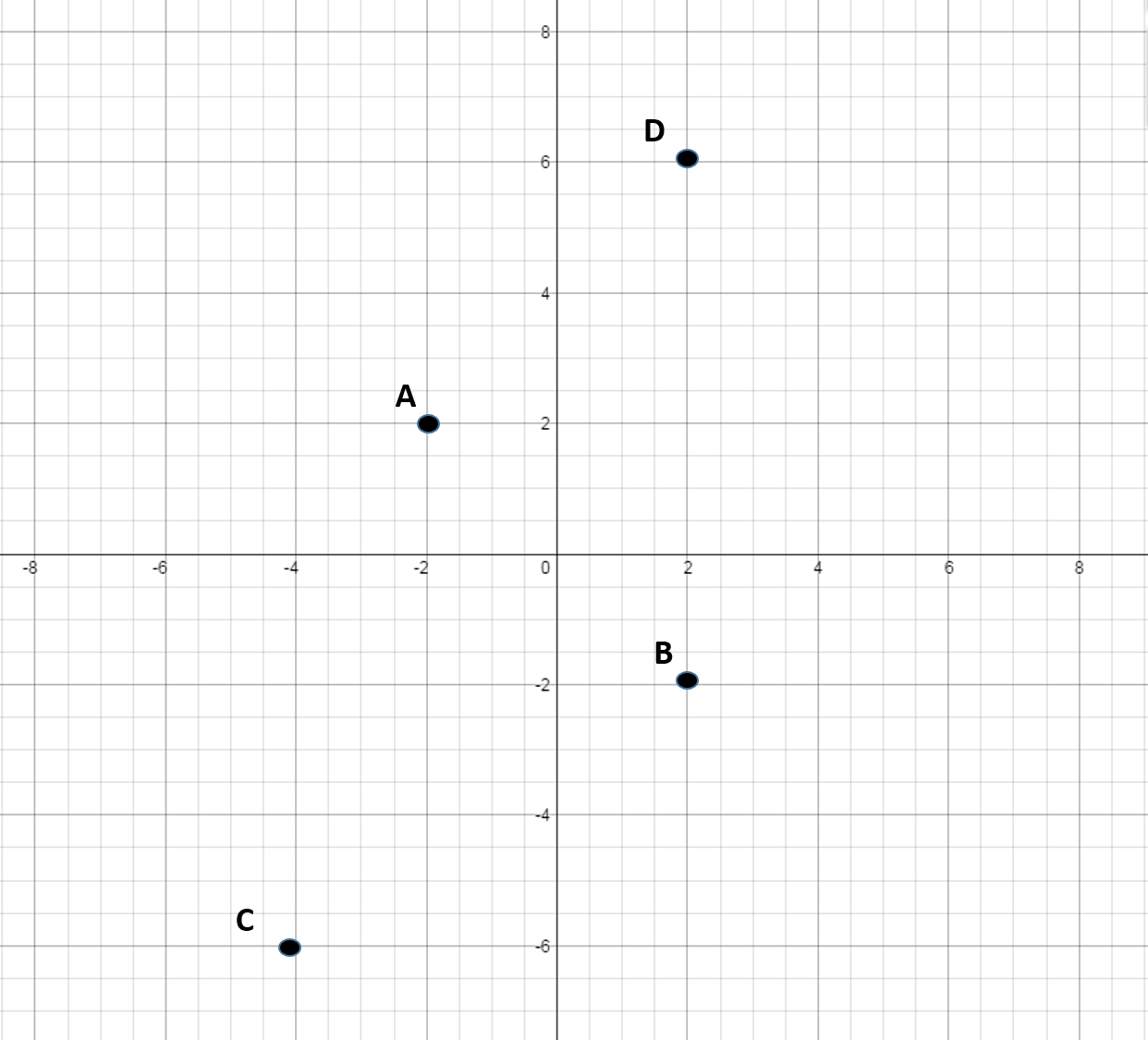C

B

A

D

D

Explanation:

To get to, move right along the x-axis by, then move up on the y-axis by.

The sign in front of each number represents which direction to go on the x or y-axis starting at the origin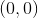. If the number is positive you go right on the x-axis or up on the y-axis. If the sign is negative then you move to the left on the x-axis or down on the y-axis.

### Example Question #2 : Graphing

Which point best represents the coordinates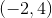?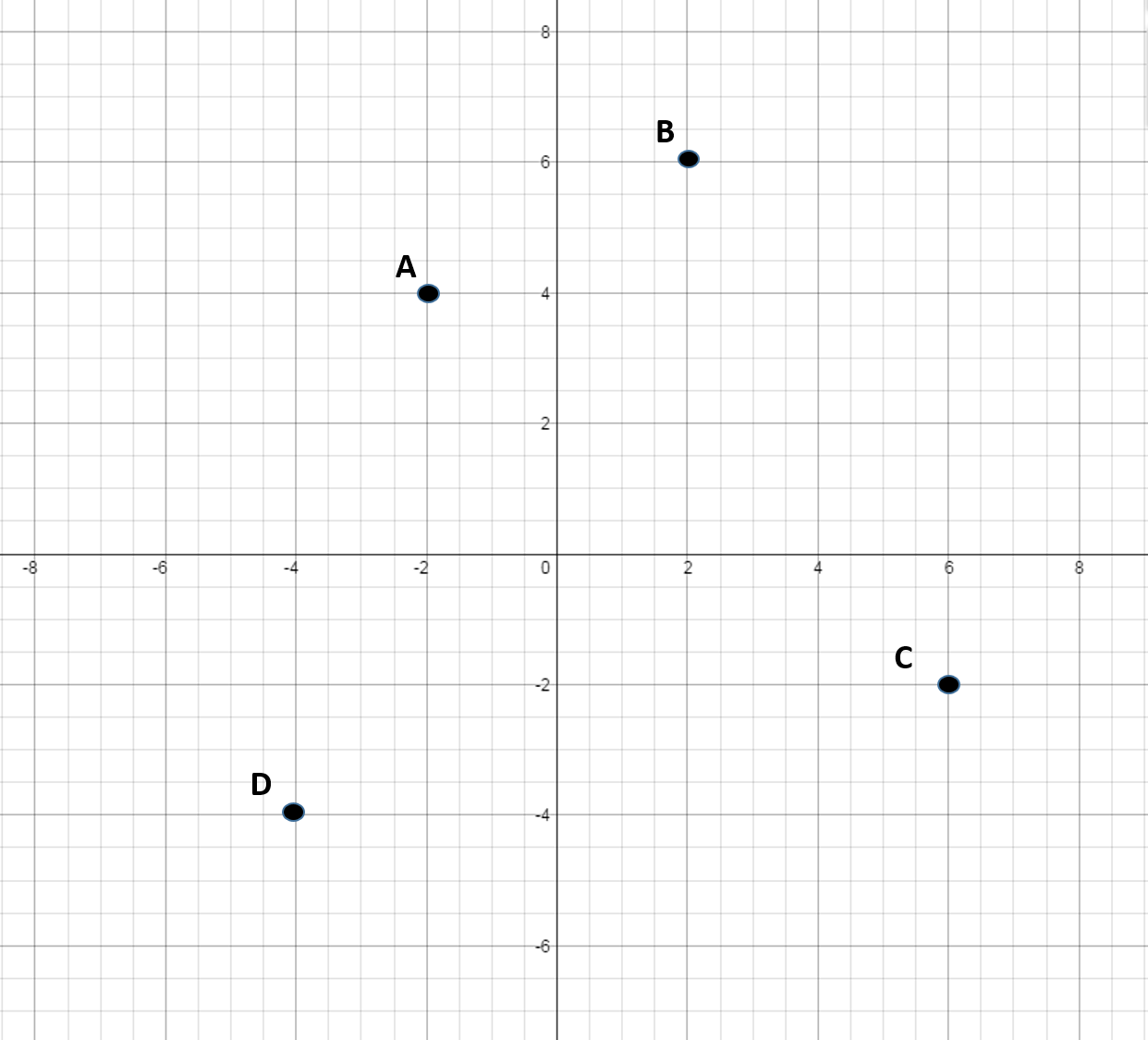A

B

D

C

A

Explanation:

The sign in front of each number represents which direction to go on the x or y-axis starting at the origin. If the number is positive you go right on the x-axis or up on the y-axis. If the sign is negative then you move to the left on the x-axis or down on the y-axis.

To get to, move left on the x-axis by, then move up on the y-axis by.

### Example Question #1 : How To Graph An Ordered Pair

Which point best represents the coordinate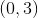?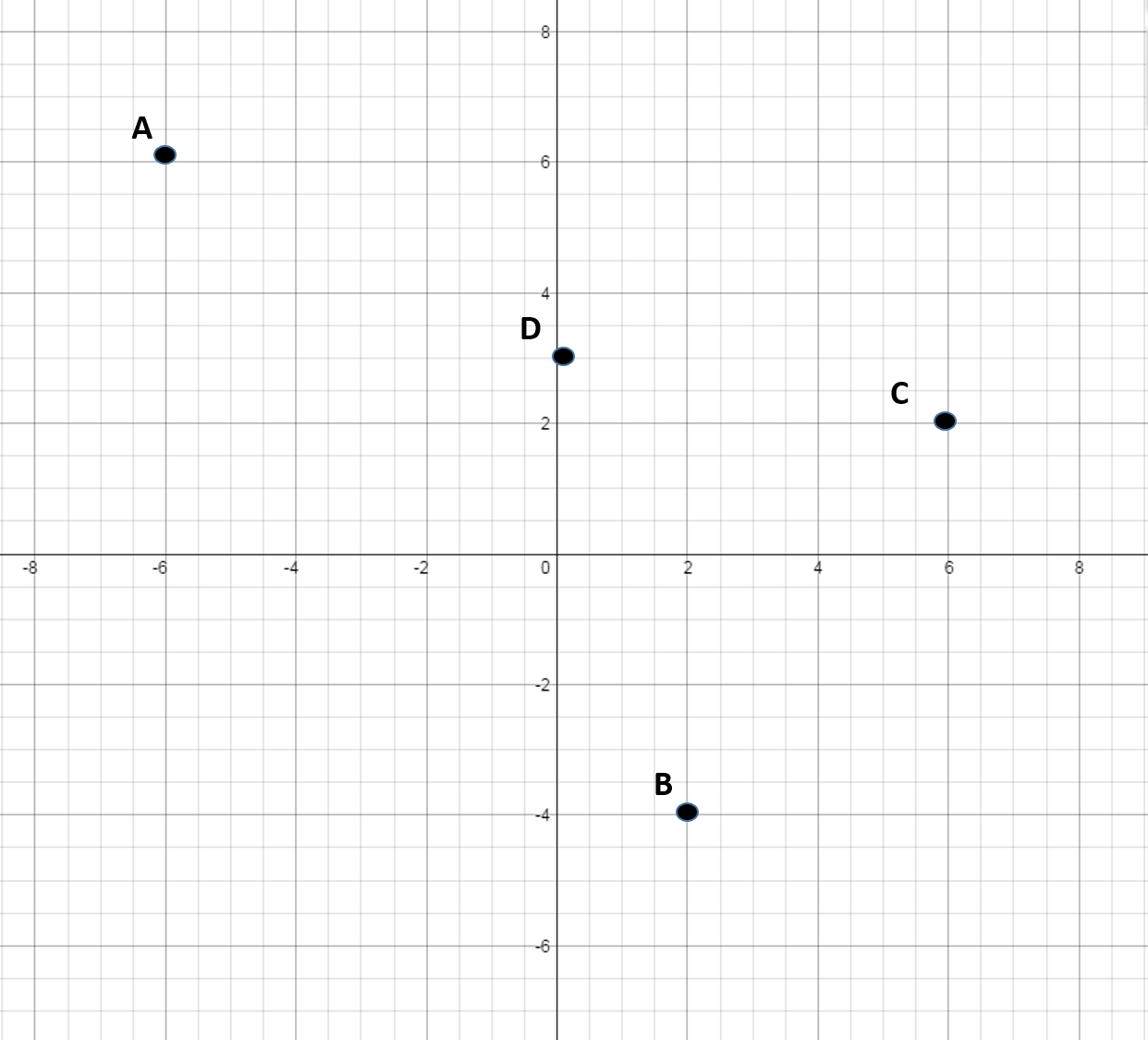D

C

A

B

D

Explanation:

The sign in front of each number represents which direction to go on the x or y-axis starting at the origin. If the number is positive you go right on the x-axis or up on the y-axis. If the sign is negative then you move to the left on the x-axis or down on the y-axis.

To get to, only move up byon the y-axis. Do not move along the x-axis because the x-coordinate is.

### Example Question #1 : How To Graph An Ordered Pair

Which point best represents the coordinate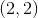?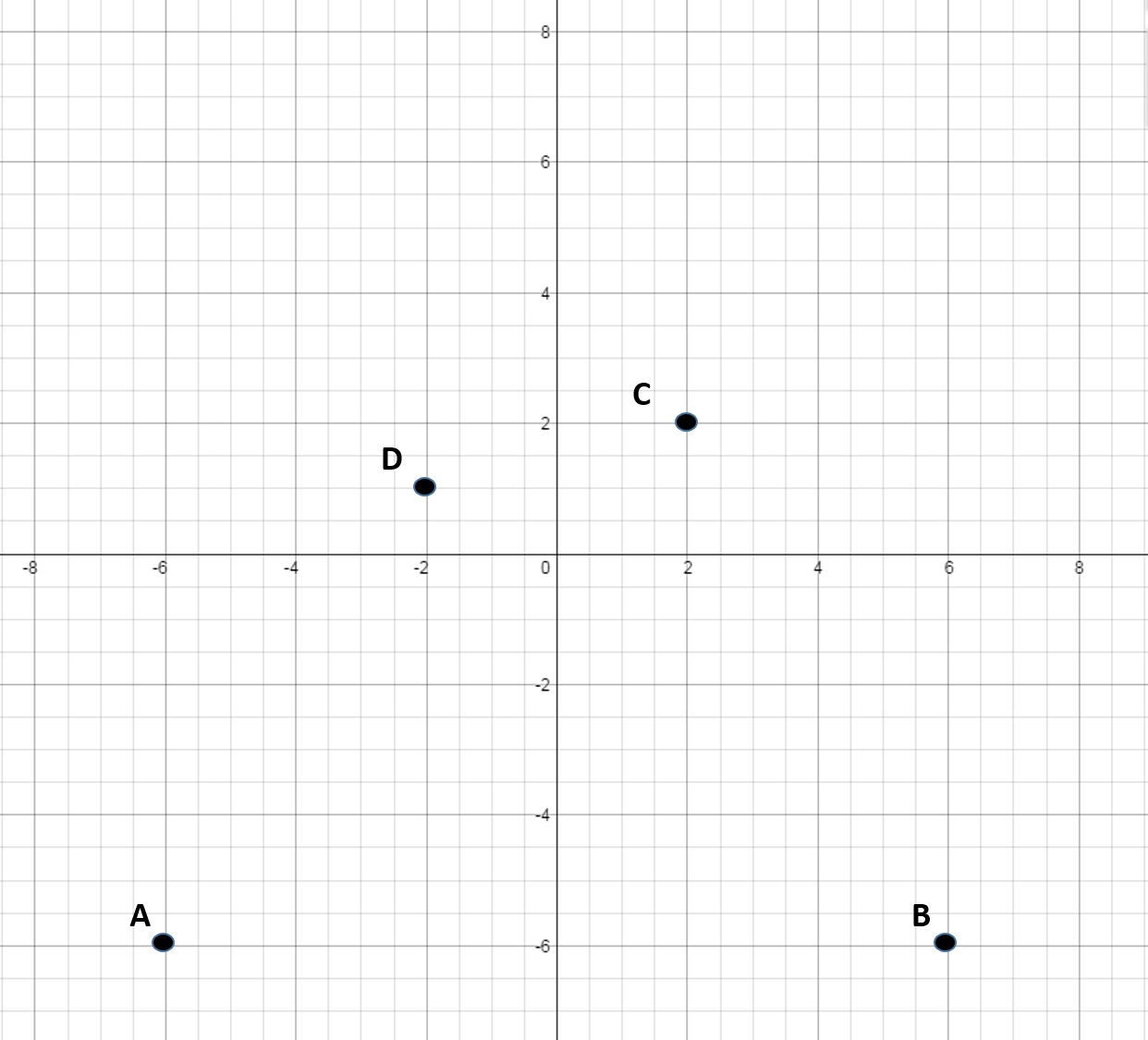A

C

B

D

C

Explanation:

To get to, move right on the x-axis bythen move up on the y-axis by.

The sign in front of each number represents which direction to go on the x or y-axis starting at the origin. If the number is positive you go right on the x-axis or up on the y-axis. If the sign is negative then you move to the left on the x-axis or down on the y-axis.

### Example Question #5 : Graphing

Which point best represents the coordinate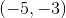?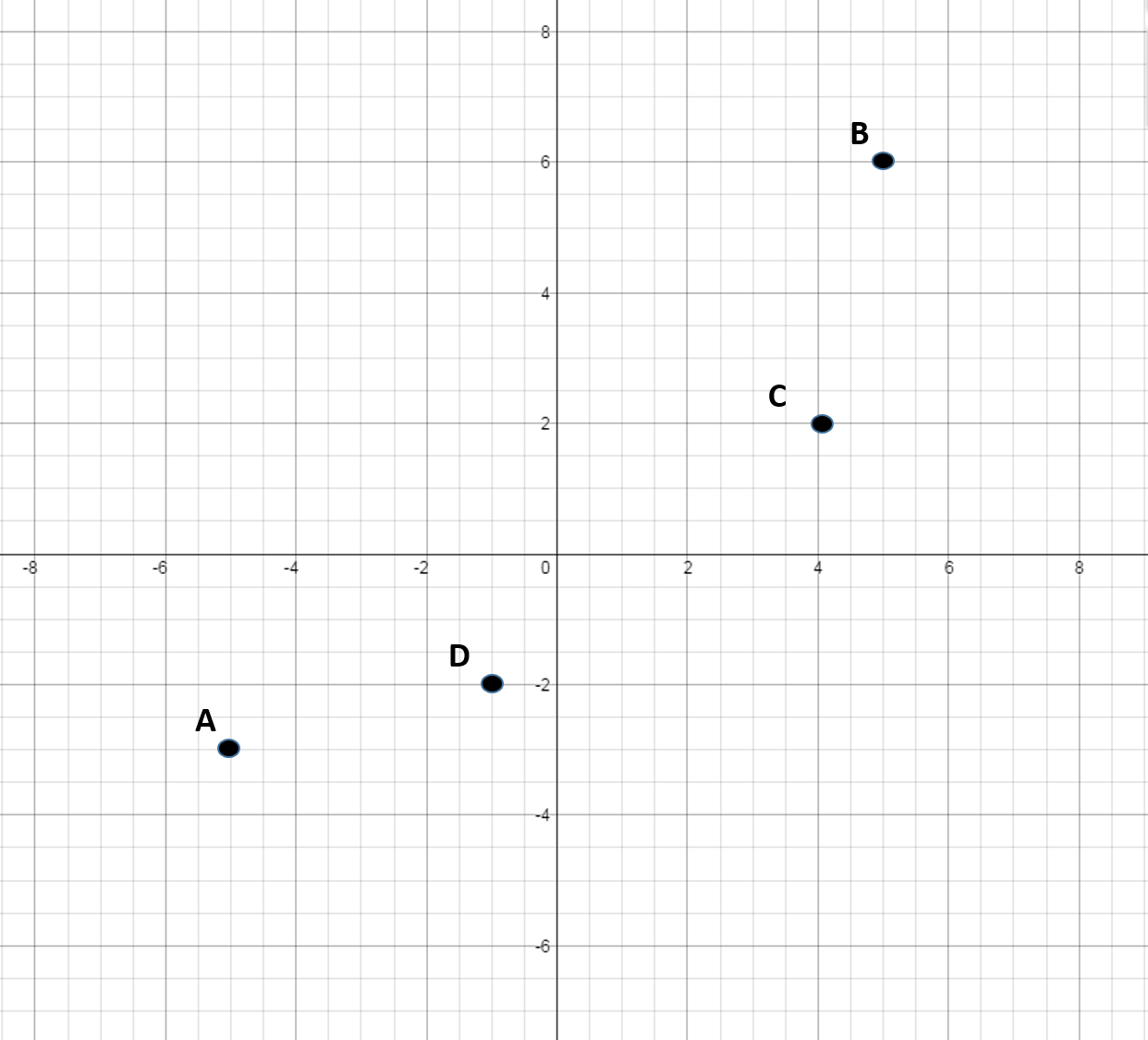C

B

D

A

A

Explanation:

To get to, move left on the x-axis bythen move down the y-axis by.

The sign in front of each number represents which direction to go on the x or y-axis starting at the origin. If the number is positive you go right on the x-axis or up on the y-axis. If the sign is negative then you move to the left on the x-axis or down on the y-axis.

### Example Question #6 : Graphing

Which point best represents the coordinate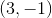?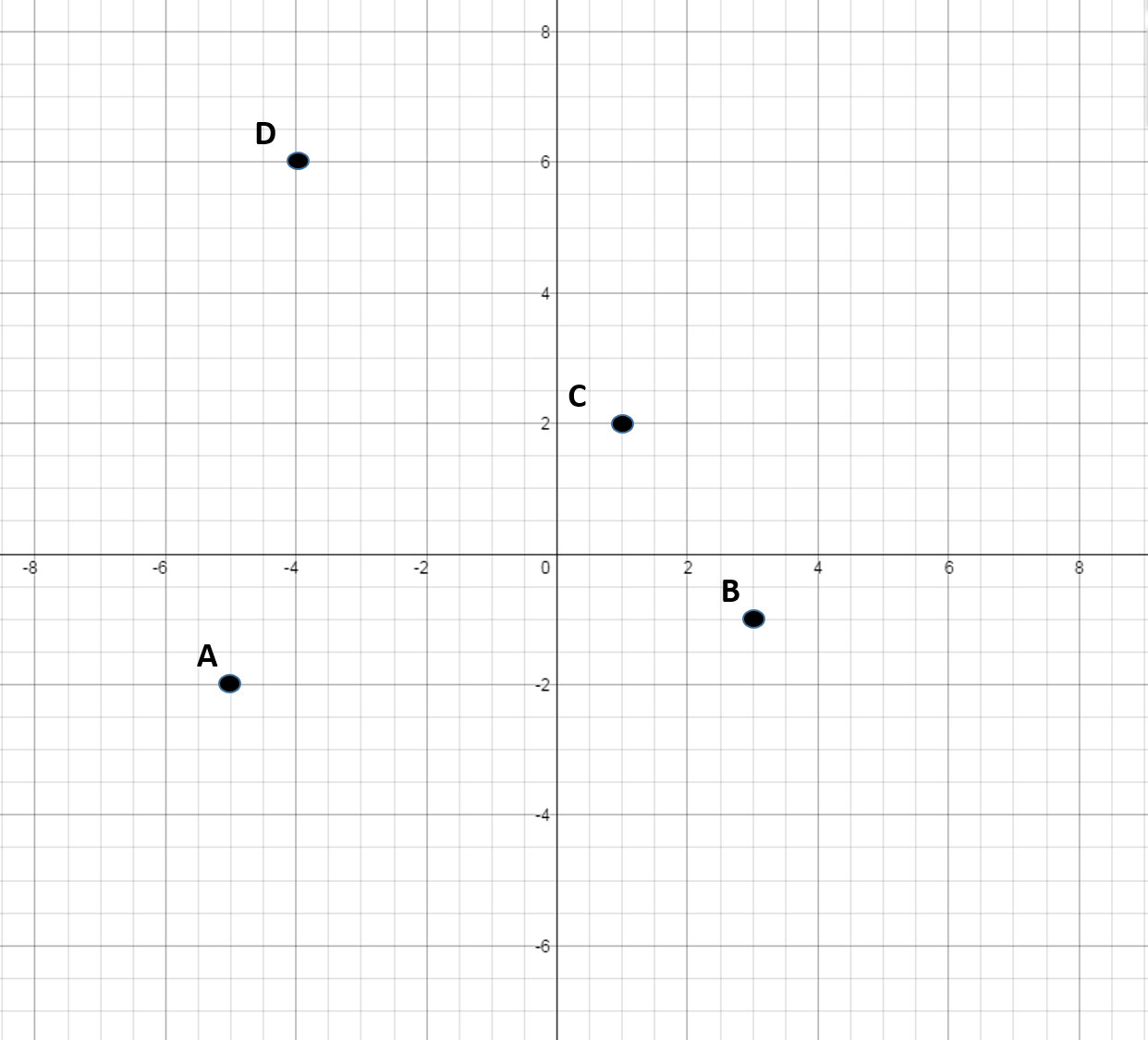A

C

B

D

B

Explanation:

The sign in front of each number represents which direction to go on the x or y-axis starting at the origin. If the number is positive you go right on the x-axis or up on the y-axis. If the sign is negative then you move to the left on the x-axis or down on the y-axis.

To get to, move right on the x-axis by, then move down on the y-axis by.

← Previous 1

### All ACT Math Resources